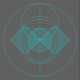This object is in archive!

# How to draw a square with 0.999... area?

gvitalie shared this question 3 years ago

Good morning, Dear GeoGebra and GeoGebrians.

How could I draw a square with area 0.999... (zero whole and nine periodic digits) or a segment with length equal 0.999... (zero whole and nine periodic digits).

I couldn't do that because GeoGebra is rounding numbers.

Thank You.2

quote: How to draw a square with 0.999... area?

.

try with 1 - 10⁻¹⁵

see attachment

Note: in the classic algebra is (a/b) b = a1

Good morning, Dear @rami.

I did it graphically, but numerically not rounding correctly I think, if I'm not wrong.

According to Pythagoras Theorem, we should sum areas of two squares something like that.

It should be 0.999..., if 2/9 + 7/9 = 0.222... + 0.777... = 0.999...1

Good morning, Dear @rami.

I've understood what's the explanation: it's difference of numerical representation with finite precision and infinite precision.

Example:

- with FINITE precision we'll always have: 1/9 + 8/9 = 0.999... until maximum precision resolution

and when we do rounding, we compensate that finite precision resolution.

- with INFINITE precision we'll always have 1/9 + 8/9 = 12

Don't you know, that 0.99999....(periodic) =1 ?

Otherwise: Tell me a number between 0.99999.... and 1.

Btw: Last digit of 6/9:

"0,66666666667 is wrong

... must be 0.66666666666"

Both results are wrong, because 0.6666666666 < 6/9 < 0.6666666667.

But 0.6666666667 is a better appoximation than 0.6666666666.